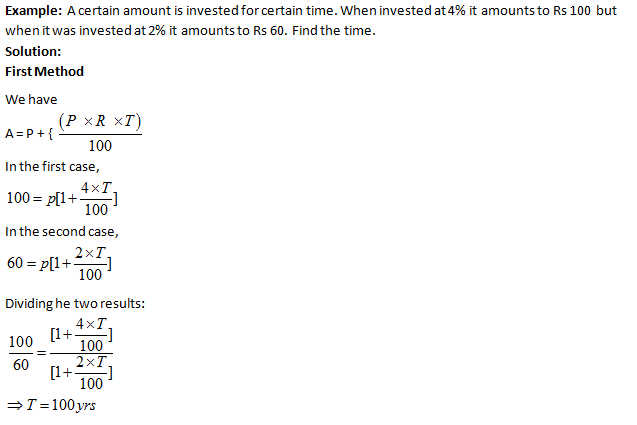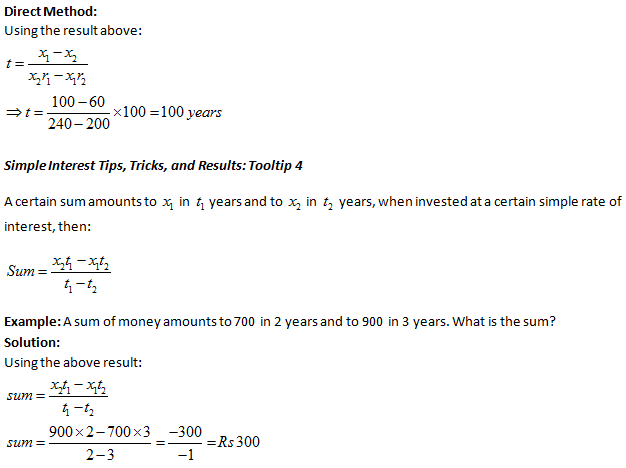Tips, Tricks& Results for Simple Interest-2

The purpose of this article is to provide you with some useful tips and tricks that you can use for Simple Interest questions. Various applications and formulas based on this concept are explained here.

Simple Interest Tips, Tricks, and Results: Tooltip 1

Change in Simple Interest when time changes from T1 to T2 is given by the formula: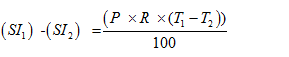Change in Simple Interest when principal changes from P1 to P2  is given by the formula: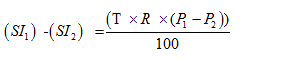Change in Simple Interest When rate of interest changes from r1to r2 is given by the formula: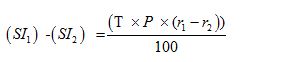Example: Simple interest on Rs 200 increases by Rs 50 when time increases by 5 years. Find rate percent per annum.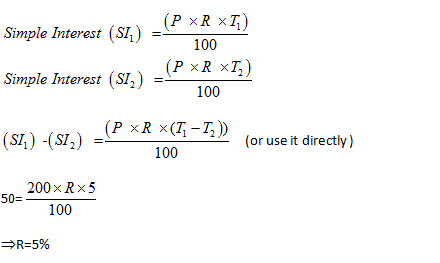Example: If the SI on Rs 1000 be more than the interest on 2000 by Rs 20 in 4 years, find the rate per annum.

Solution: When principal changes from  P1to P2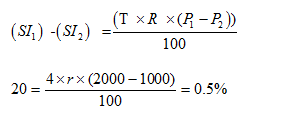Simple Interest Tips, Tricks, and Results: Tooltip 2

If a person deposits A1  amount at r1% per annum and amount A2 at r2% per annum, then the rate of interest for the whole sum is {(A1r1 + A2r2)/(A1+A2)}

Example: A man deposits Rs.1000 at 4% per annum and Rs 2000 at 5% per annum, find the rate of interest for the whole sum.

Solution:
Method-1:
Using the formula for, Simple Interest (SI) =  {(P x R x T)/100}
X = {(1000 x 4 x 1)/100} = 40
Y = (2000 x 5 x 1)/100 = 100
Total interest = 100+40=Rs. 140
Total principal =1000+2000=Rs.3000
Using the formula:
140 = {(3000 x R x 1)/100}
R = 14/3%

Method-2:
Applying above concept {(A1r1 + A2r2)/(A1+A2)} , the rate of interest is
[{(1000 x 4) + (2000 x 5)}/(1000 + 2000)]
= 14000/3000
14/3%

Simple Interest Tips, Tricks, and Results: Tooltip 3
A certain amount is invested for certain time but at different rates. When rate per annum is r1 the amount becomes x1 but at rate  r2 per annum, the amount becomes x2 .
Time, t, is given by the formula:
t = {(x1 – x2)/(x2r1 – x1r2)}*100